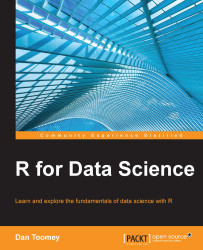•#### R for Data Science#### Overview of this book

R for Data ScienceCreditswww.PacktPub.comPrefaceFree Chapter
Data Mining PatternsData Mining SequencesText MiningData Analysis – Regression AnalysisData Analysis – CorrelationData Analysis – ClusteringData Visualization – R GraphicsData Visualization – PlottingData Visualization – 3DMachine Learning in ActionPredicting Events with Machine LearningSupervised and Unsupervised LearningIndex## Bar charts and plots

In this section, I will show you how to generate bar charts and bar plots using R. I think whether you call a particular graphic a bar chart or a bar plot is up to you. There are minor differences between bar charts and bar plots. In both cases, we have bars representing counts; we display them across your graphic. The steps (and results) involved are similar.

### Bar plot

R programming allows us to create bar charts in a variety of ways. The standard function in R is the `barplot` function. The `barplot` function only requires a list of the heights to be displayed. All of the following parameters are optional.

#### Usage

The `barplot` function is used as follows:

`> barplot(data)`

The various parameters are as follows:

```        height, width = 1, space = NULL,
names.arg = NULL, legend.text = NULL, beside = FALSE,
horiz = FALSE, density = NULL, angle = 45,
col = NULL, border = par("fg"),
main = NULL, sub = NULL, xlab = NULL, ylab = NULL,
xlim = NULL...```